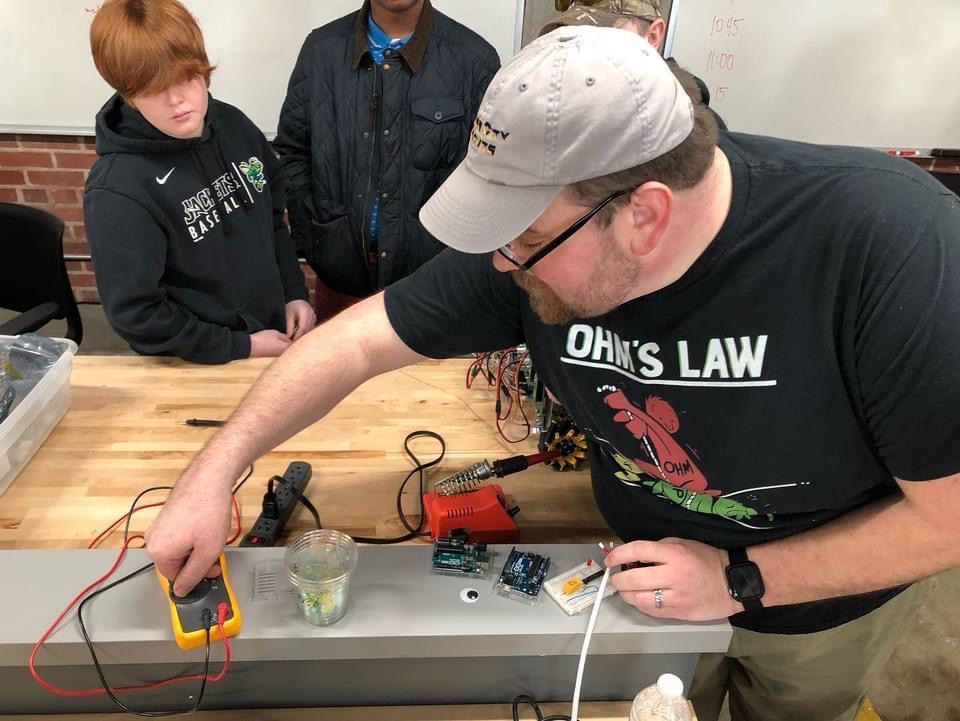Sales@CyberCityCircuits.com

# Electricity 101: Understanding Voltage, Current, and ResistanceWhen we talk about electricity, there are a few key units of measurement that we use to describe how it flows and how it is used. These units are voltage, current, and resistance. Let’s take a closer look at each of these:

## Voltage

Voltage, also known as electric potential difference, is a measure of the force that pushes electricity through a conductor. It is measured in units called volts (V). The higher the voltage, the stronger the force pushing the electricity through the conductor.

## Current

Current is a measure of the flow of electricity through a conductor. It is measured in units called amperes, or amps (A). The higher the current, the more electricity is flowing through the conductor.

## Resistance

Resistance is a measure of how hard it is for the electricity to flow through a conductor. It is measured in units called ohms (Ω). The higher the resistance, the harder it is for the electricity to flow.

We can use these three units of measurement to understand how electricity behaves in different situations. For example, we can use Ohm’s Law to calculate the current in a circuit if we know the voltage and the resistance. Ohm’s Law states that:

## Current (I) = Voltage (V) / Resistance (R)

Imagine you have a small portable fan that runs on electricity. The battery in the fan has a voltage of 4.5 volts and the motor that powers the fan has a resistance of 1.5 ohms. Using Ohm’s Law, we can calculate that the current flowing through the motor will be 3 amps (4.5 volts / 1.5 ohms = 3 amps).

In this example, the voltage of the battery is providing the force to push the electricity through the conductor (the motor), and the resistance of the motor determines how easily the electricity can flow. The current, measured in amps, tells us how much electricity is flowing through the motor.

Understanding these basic units of measurement is important for working with electricity and designing electrical circuits. It helps us predict how electricity will behave and how different devices and circuits will work.

So the next time you’re working with electricity, remember these key units of measurement and how they help us understand the flow of electricity!

Posted in